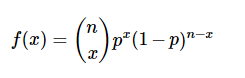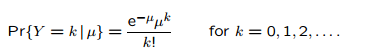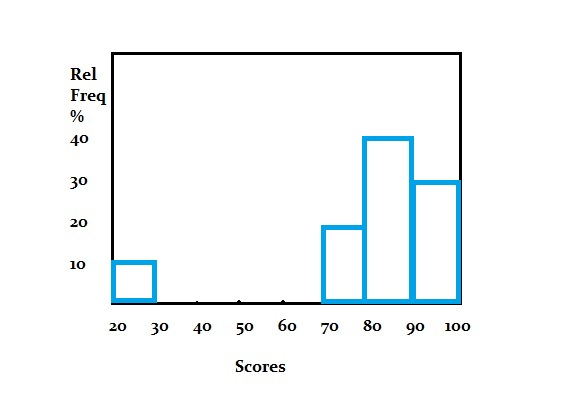# Probability Mass Function (PMF): Definition, Examples

Contents:

## What is a Probability Mass Function?

Watch the video for an overview and a few examples, or read on below:

A probability mass function (PMF)— also called a frequency function— gives you probabilities for discrete random variables. “Random variables” are variables from experiments like dice rolls, choosing a number out of a hat, or getting a high score on a test. The “discrete” part means that there’s a set number of outcomes. For example, you can only roll a 1, 2, 3, 4, 5, or 6 on a die.

Its counterpart is the probability density function, which gives probabilities for continuous random variables.

A PMF can be an equation, a table, or a graph.

## Probability Mass Function Equations: Examples

A PMF equation looks like this:

P(X = x).

That just means “the probability that X takes on some value x”.
It’s not a very useful equation on its own; What’s more useful is an equation that tells you the probability of some individual event happening. For example:

P(X = 1) = 0.2 * 0.2.

How you come up with these equations depends mostly on what type of event you have. For example, the binomial distribution PMF is:And the Poisson distribution PMF is:Need help with a homework question? Check out our tutoring page!

## Tables and Graphs

The histogram is just a graph of a PMF.On the x-axis are the discrete random variables; On the y-axis are the probabilities for each discrete variable. The area under a curve of a probability mass function is 100% (i.e. the probability of all events, when added together, is 100%). The above histogram shows:

• 10% of people scored between 20 and 30,
• 20% of people scored between 70 and 80,
• 40% of people scored between 80 and 90, and
• 30% of people scored between 90 and 100.

That gives a total of 10% + 20% + 40% + 30% = 100%.

Another example:
The following table and graph show the PMF for an experiment. Note how all of the probabilities add up to 1:## Formal Definition

Like many mathematical terms, there’s the informal definition (given above), and then there’s the formal one:

The probability mass function, f(x) = P(X = x), of a discrete random variable X has the following properties:

1. All probabilities are positive: fx(x) ≥ 0.
2. Any event in the distribution (e.g. “scoring between 20 and 30”) has a probability of happening of between 0 and 1 (e.g. 0% and 100%).
3. The summation of all probabilities is 100% (i.e. 1 as a decimal): Σfx(x) = 1.
4. An individual probability is found by adding up the x-values in event A. P(X Ε A) =5. Where Σ is summation notation.

## Terminology note

The general use of the term PMF means a probability distribution for a discrete random variable. However, some authors (not many) use the term “probability mass function” to mean either a discrete or continuous probability distribution. To add to the confusion, other authors might call a PMF a probability function or frequency function. Make sure you know the author’s intent when reading a textbook.

## References

Everitt, B. S.; Skrondal, A. (2010), The Cambridge Dictionary of Statistics, Cambridge University Press.
Vogt, W.P. (2005). Dictionary of Statistics & Methodology: A Nontechnical Guide for the Social Sciences. SAGE.

CITE THIS AS:
Stephanie Glen. "Probability Mass Function (PMF): Definition, Examples" From StatisticsHowTo.com: Elementary Statistics for the rest of us! https://www.statisticshowto.com/probability-mass-function-pmf-definition-examples/